# MATHS PATENT: THE ESSENTIALS

The essentials to know for the mathematics test for the college diploma.

I. FRACTIONS:

A fraction looks like this, where a is the numerator and b is the denominator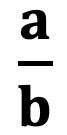I.1. Addition or subtraction of fractions

Method :

• reduce to the same denominator,
• add or subtract the numerators and keep the common denominator.

Example:12 is the common denominator, 12 is a multiple of 6, we transform a single fraction

I.2. Multiplication of fractions

Method : Multiply the numerators between them as well as the denominators between them (do not reduce to the same denominator)

Example:I.3. Division of fractions:

Method : multiply the first fraction by the inverse of the second fraction

Example:

Priority rules :

First perform the calculations in brackets then inside the brackets the parentheses starting with the multiplications and divisions (from left to right) then additions and subtractions (from left to right).

ou CPMDAS or Brackets / Parentheses / Multiplication / Division / Addition / Subtraction

Example: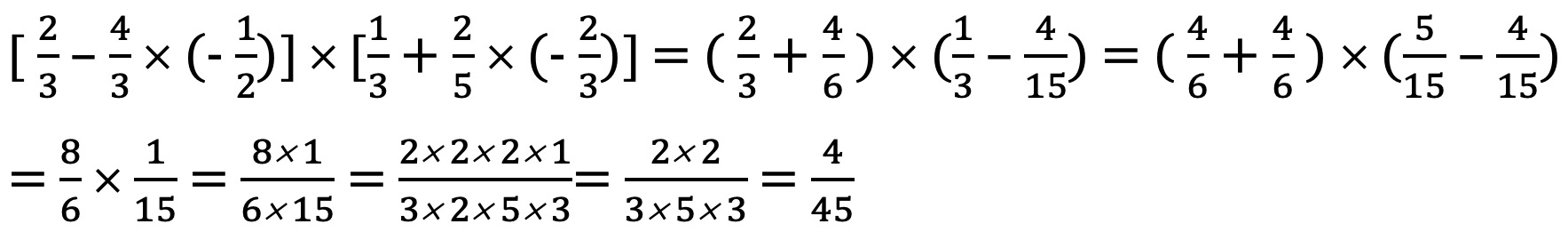Think about reducing fractions to their simplest expression !

II. POWERS:

II.1. Basic calculation rules: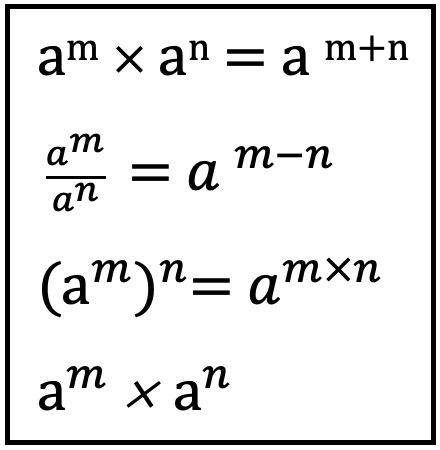where a, b, m and n are relative integers

II.2. Powers of 10:

To calculate with powers of 10, group the powers of 10 and the numbers other than the powers

Example:II.3. Power of a number:

Same method as with powers of 10

Example: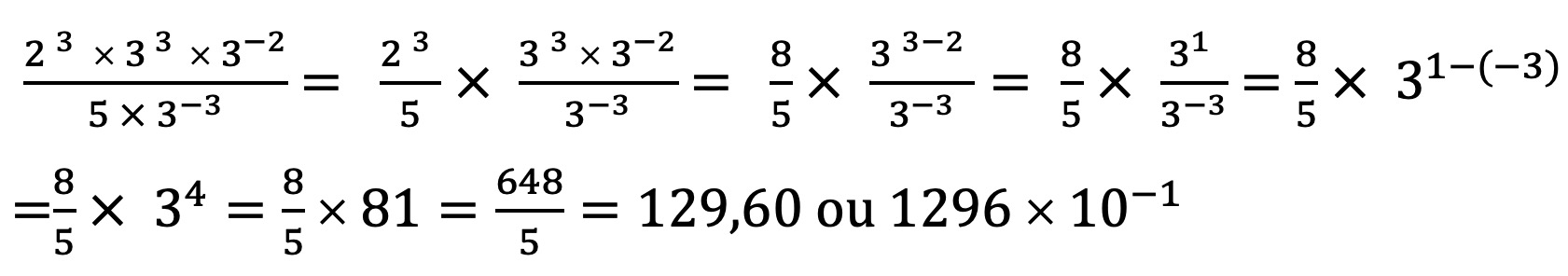III. SQUARE ROOTS:

III. 1. Definition:

La square root a positive number a, is the positive number whose square is equal to a.

We note it. The signis called radical.

III.2. Basic formulas: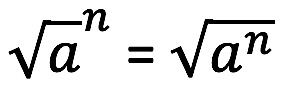IV. FUNCTIONS :

IV. 1. Linear function:

fonction linear is a function of the form f: x -> ax where a is a real number called the coefficient of the linear function or the coefficient of proportionality.

Property
The graphical representation of a linear function is a straight line that passes through the origin of the coordinate system.
si a> 0, then the right “goes up”.
si a <0, then the line "goes down".

Special cases
- An increase in p% results in the linear function f (x) = (1 + p / 100) x
- A reduction of p% results in the linear function f (x) = (1 - p / 100) x

IV. 2. Affine function:

linear function is a function of the form f: x -> ax + b where a is a real number called the coefficient of the linear function or coefficient of proportionality, and b the y-intercept.

Property
The graphical representation of a linear function is a line.
si a > 0, then the right “goes up”.
si a <0, then the line "goes down".

Special cases
A linear function is a special case of an affine function
.

V. CALCULATION OF A LENGTH :

V. 1. Right triangle:

Reminder of the Pythagorean theorem : the square of the hypotenuse (side opposite to the right angle) is equal to the sum of the squares of the other two sides.

In a triangle ABC right at B, AC being the hypotenuse, where AB = b, BC = b and AC = c:

AB2 + BC2 = AC2 or even  a2 + b2 = c2

The Pythagorean theorem thus makes it possible to calculate the length of one of the sides of a right triangle if we know the other two.

The converse of the Pythagorean theorem : a triangle is right-angled if and only if the square of the length of the longest side is equal to the sum of the squares of the lengths of the other two sides.

This theorem is used to to prove that a triangle is right-angled when we know the lengths of its three sides.

Trigonometry reminder :

The Sinus is equal to the length of the Opposite side divided by the length of the Hypotenuse (SOH). The Cosine is equal to the length of the Adjacent side divided by the length of the Hypotenuse (CAH). Tangent is equal to the length of the Opposite side divided by the length of the Adjacent side (TOA).

Mnemonic: SOHCAHTOA

For any acute angle â of a right triangle:

(cos â)2 + (sin â)2 = 1 et tan â = sin â / cos â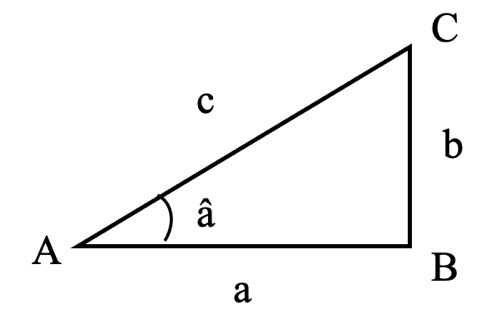Example:

AC is the hypotenuse and BC the opposite, the triangle being right at B,

sin â = BC / AC i.e. sin 40 = BC / 6 hence BC = sin 40 x 6 = 4,47 cm

V.2. In any triangle:

Reminder of Thales' theorem : if two parallel lines intersect two secant lines then they determine two triangles whose corresponding sides have proportional lengths.

Two configurations are found:

Example:

Calculate AB

DE // AB and EA and DB are secant in C. According to Thales' theorem,

VI. CALCULATION OF ANGLES:

VI. 1. Any triangle:

Properties :

• The sum of the angles of a triangle being equal to 180 ° C, if we know the value of 2 of the 3 angles we can deduce the third.
• Two corresponding or alternate internal angles (located on either side of the secant, between the two lines and not adjacent.) Are equal if the lines are parallel.

.• Two angles opposed by the vertex are equal.

VI.2. Rectangle triangle :

Use of trigonometry:

Examples : Calculation of the angle â

AB is the adjacent side and BC the opposite side of the angle â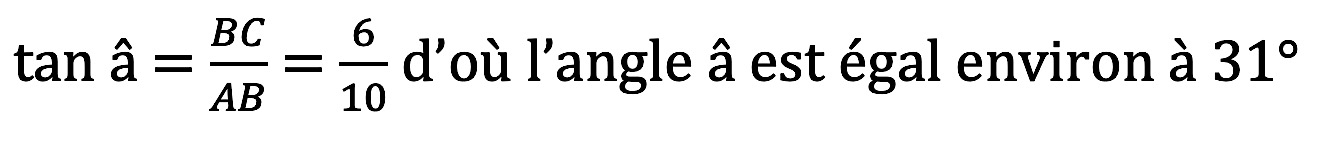VI.3. Inscribed angles and central angles:

properties:

• The inscribed angle theorem says if two angles inscribed in a circle intercept the same arc then they have the same measure.
• The central angle theorem affirm that, in a circle, a central angle is twice an inscribed angle intercepting the same arc.VII. PARALLEL AND PERPENDICULAR RIGHTS:

VII.1. Properties:

• If two lines are perpendicular to the same third, then they are parallel to each other.
• If two lines are parallel, then any perpendicular to one is perpendicular to the other.
• If two lines are parallel to the same third then they are parallel to each other.

VII.2. Right triangle demonstration or not:

If we know the dimension of the 3 sides, we calculate the square of the larger side and the sum of the squares of the 2 other sides.

• If the sum is equal, we deduce that the triangle is rectangles (Pyhagorean theorem).
• If the sum is different, we conclude that the triangle is not rectangle (contraposed of the Pythagorean theorem).

Rappel : if the theorem is the following If we have A then we get B. The reciprocal of this theorem would be "If we B then we get A (not always true) and the contraposed : if we don't have B then we don't get A (always true).

VII.3. Triangle inscribed in a circle:

If a triangle is right-angled then the center of its circumscribed circle is the midpoint of its hypotenuse and the median relative to the hypotenuse is half that of the hypotenuse.If a triangle has for vertices the ends of a diameter and a point of the circle then it is rectangle at this point.

VII.4. Prove that 2 lines are parallel or not:

If we know 4 lengths in configuration of Thales.

VIII. DEVELOPMENT OF EXPRESSIONS

VIII.1. The double distributive rule:

Example:

(2x - 3) (4+ 5x) = 8x + 10 x2 -12 - 15x = 10 x2 -7x - 12

VIII.2. Remarkable identities:

• (a + b)2 = A2 + 2ab + b2
• (a - b)2 = A2 - 2ab + b2
• (a + b) (a -b) = a2 - b2

Examples:

(x + 2)2 = x2 + 2x + 4 (3x - 2)2 = 9x2 - 6x + 4 (2x + 3) (2x -3) = 4x2 - 9

IX. INVOICING OF EXPRESSIONS

IX.1 A common factor:

Example: (4x - 3)2 - (4x -2) (4x -3) = (4x-3) ((4x -3) - (4x -2)) = (4x - 3) (-1) = 3 - 4x

IX.2. Use of remarkable identities:

• Find the double product of type (.. + ..)2 or (..- ..)2

Example: x2 -6x + 9 = (x - 3)2 check the double product 2 x (-3) = - 6x to arrive at the result

• Look for a common factor

Example: 9 - 36x2 = (3 + 6x) (3 - 6x)

X. SPATIAL GEOMETRY

X.1. Formulas on areas

X.2. Formulas on volumes:

X.3. Formulas on the areas of solids:

Air lateral = sum of the areas of the lateral facess

Air total = Area lateral + Area basis (for a right prism, the side faces are rectangles and the bases are two identical polygons)

Air sphere = 4 × π × R2

X.4. Sections:

k = HO '/ HO = HA' / HA = HB '/ HB = HC' / HC

Properties linked to an enlargement of coefficient k:

• lengths are multiplied or divided by k
• the areas are multiplied by k2
• the volumes are multiplied by k3

Quote on Mathematics:

“In mathematics, 'obvious' is the most dangerous word.”

Eric temple bell

Similar article:

Physics patent: the essentials